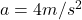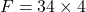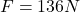How much force is needed to accelerate a 34 kg skier at 4 m/s2?

Question

How much force is needed to accelerate a 34 kg skier at 4 m/s2?

in progress 0
6 months 2021-08-06T09:42:40+00:00 1 Answers 9 views 0

Net force needed to accelerate the skier is 136 N

Explanation:

As per Newton’s 2nd law we know that net force on the system is product of mass and its acceleration

here we know that

m = 34 kgso we have

F = ma